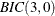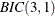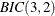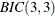### The MINIC Method

The minimum information criterion (MINIC) method can tentatively identify the order of a stationary and invertible ARMA process. Note that Hannan and Rissannen (1982) proposed this method, and Box, Jenkins, and Reinsel (1994) and Choi (1992) provide useful descriptions of the algorithm.

Given a stationary and invertible time series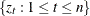with mean corrected form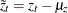with a true autoregressive order of
and with a true moving-average order of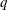, you can use the MINIC method to compute information criteria (or penalty functions) for various autoregressive and moving average orders. The following paragraphs provide a brief description of the algorithm.

If the series is a stationary and invertible ARMA(p, q ) process of the form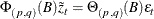the error series can be approximated by a high-order AR process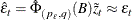where the parameter estimates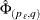are obtained from the Yule-Walker estimates. The choice of the autoregressive order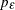is determined by the order that minimizes the Akaike information criterion (AIC) in the range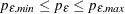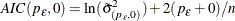where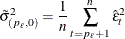Note that Hannan and Rissannen (1982) use the Bayesian information criterion (BIC) to determine the autoregressive order used to estimate the error series. Box, Jenkins, and Reinsel (1994) and Choi (1992) recommend the AIC.

Once the error series has been estimated for autoregressive test order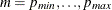and for moving-average test order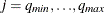, the OLS estimates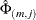and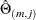are computed from the regression model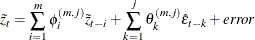From the preceding parameter estimates, the BIC is then computed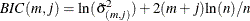where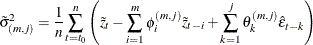where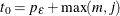.

A MINIC table is then constructed using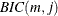; see Table 7.6. If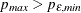, the preceding regression might fail due to linear dependence on the estimated error series and the mean-corrected series. Values ofthat cannot be computed are set to missing. For large autoregressive and moving-average test orders with relatively few observations, a nearly perfect fit can result. This condition can be identified by a large negativevalue.

Table 7.6: MINIC Table

 MA AR 0 1 2 30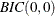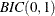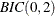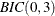1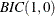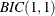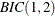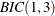2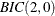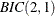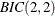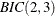3